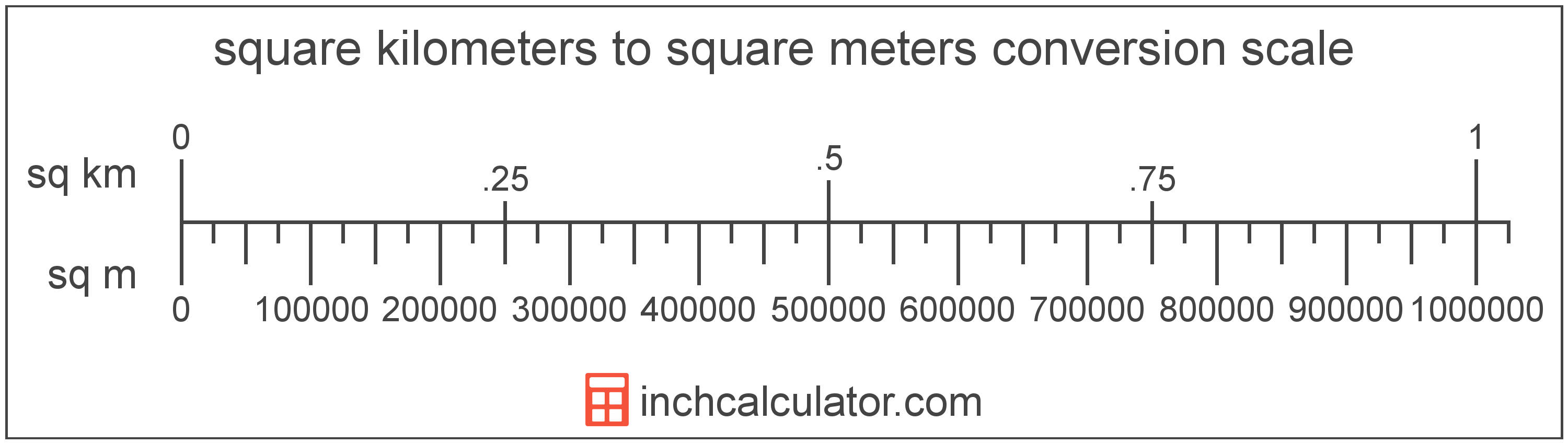# Square Meters to Square Kilometers Converter

Enter the area in square meters below to get the value converted to square kilometers.

(find square meters)
Results in Square Kilometers:1 sq m = 1.0E-6 sq km
Hint: use a scientific notation calculator to convert E notation to decimal

Do you want to convert square kilometers to square meters?

## How to Convert Square Meters to Square Kilometers

To convert a measurement in square meters to a measurement in square kilometers, divide the area by the following conversion ratio: 1,000,000 square meters/square kilometer.

Since one square kilometer is equal to 1,000,000 square meters, you can use this simple formula to convert:

square kilometers = square meters ÷ 1,000,000

The area in square kilometers is equal to the area in square meters divided by 1,000,000.

For example, here's how to convert 5,000 square meters to square kilometers using the formula above.
square kilometers = (5,000 sq m ÷ 1,000,000) = 0.005 sq km## What is a Square Meter?

One square meter is equivalent to the area of a square with sides that are each 1 meter in length. One square meter is equal to 10.76391 square feet or 10,000 square centimeters.

The square meter, or square metre, is the SI derived unit for area in the metric system. A square meter is sometimes also referred to as a square m. Square meters can be abbreviated as sq m, and are also sometimes abbreviated as . For example, 1 square meter can be written as 1 sq m or 1 m².

You can use a square meters calculator to calculate the area of a space if you know its dimensions.

## What is a Square Kilometer?

One square kilometer is equivalent to the area of a square with sides that are each 1 kilometer in length. One square kilometer is equal to 0.386102 square miles or 1,000,000 square meters.

The square kilometer, or square kilometre, is a multiple of the square meter, which is the SI derived unit for area. In the metric system, "kilo" is the prefix for thousands, or 103. A square kilometer is sometimes also referred to as a square km. Square kilometers can be abbreviated as sq km, and are also sometimes abbreviated as km². For example, 1 square kilometer can be written as 1 sq km or 1 km².

## Square Meter to Square Kilometer Conversion Table

Table showing various square meter measurements converted to square kilometers.
Square Meters Square Kilometers
1 sq m 0.000001 sq km
2 sq m 0.000002 sq km
3 sq m 0.000003 sq km
4 sq m 0.000004 sq km
5 sq m 0.000005 sq km
6 sq m 0.000006 sq km
7 sq m 0.000007 sq km
8 sq m 0.000008 sq km
9 sq m 0.000009 sq km
10 sq m 0.00001 sq km
100 sq m 0.0001 sq km
1,000 sq m 0.001 sq km
10,000 sq m 0.01 sq km
100,000 sq m 0.1 sq km
1,000,000 sq m 1 sq km

## References

1. Collins Dictionary, Definition of 'square meter', https://www.collinsdictionary.com/us/dictionary/english/square-meter